# 2nd PUC Electronics Question Bank Chapter 12 C Programming

Students can Download 2nd PUC Electronics Chapter 12 C Programming Questions and Answers, Notes Pdf, 2nd PUC Electronics Question Bank with Answers helps you to revise the complete Karnataka State Board Syllabus and score more marks in your examinations.

## Karnataka 2nd PUC Electronics Question Bank Chapter 12 C Programming

### 2nd PUC Electronics C Programming One Mark Questions and Answers

Question 1.
What is C programming language?
C is a powerful, flexible, portable and structured programming language.

Question 2.
Why is C programming language suitable for both systems and applications programming?
C programming combines the features of high level language with the elements of assembler, hence it is suitable for both systems and applications programming.

Question 3.
What is a Function?
A Function is a subroutine that may include one or more statements designed to perform a specific task.Question 4.
What is a C pre-processor?
C pre-processor is a program that processes the source code before it passes through the compiler.

Question 5.
What is debugging a C program?
The process of detecting and correcting errors in the program is debugging.

Question 6.
What is a syntax error?
The syntax errors are due to the violation of syntax (grammar rules of programming languge).

Question 7.
What is a logical error?
Logical errors occur during the coding process, when the type of the data is wrongly selected and is used for computation. [ int a,b,sum; printf(“\n enter the two numbers”); scanf(“%d%d ”,&a,&b);sum=a+b;printf(“\nsum is%d”,b);]Question 8.
What is run time error?
Run time errors occur when the user tries to run ambiguous instriictions.
(like infinite loop, divided by 0 etc)

Question 9.
What is testing a program?
The process of executing the program to test the correctness of the results is testing.

Question 10.
Where does the program be executed in a Computer?
Program is executed in the central processing unit(CPU).

Question 11.
What are C tokens?
The basic and smallest units of a C program are C tokens.(keywords, constants, operators etc)

Question 12.
What are constants?
The quantity which does not change during the execution of a program is a constant.

Question 13.
Write the syntax for assigning values to variables.
The syntax for assigning value to a variable is variable_name = value; (a=5;)Question 14.
Write the range of values for a keyword int.
Range for int is from (-2-16) to (216-1) i.e. -32768 to 32767

Question 15.
Write the ASCII value for the character constant”=”.
ASCII value of“=” is 061

Question 16.
What is a statement?
Statement is an instruction to a computer to perform a specific operation.

Question 17.
Write the arithmetic operator used to represent remainder obtained in integer division.
% (modulus) operator is used to represent the remainder obtained in integer division.

Question 18.
What is an operator?
Operator is a symbol that tells the computer to perform certain arithmetic or logical operations.

Question 19.
What is the value of 17%-2?
The value of 17%-2 is +1

Question 20.
What is a relational operator?
Relational operator is used to compare two operands in an expression.Question 21.
What is the meaning of the symbol”~”?
is 1 ’s complement operator.

Question 22.
Write the symbol of exclusive-OR operation.
Symbol for Exclusive -OR operation is “Λ”(caret).

Question 23.
If A = 1, and B=0, what is the value of A Λ B?
1 Λ 0 = 1 (1 XOR 0 = 1).

Question 24.
What is the difference between “=” and “= =”?
“=” is an assignment operator whereas “= =” is an arithmetic operator.

Question  25.
What is the description of the following operator “Λ=”?
Λ=” represents bitwise XOR assignment operator. ( c=c Λ b or c Λ =b;)

Question 26.
Where is a conditional operator used?
Conditional operator is used to test the relation between two variables.

Question 27.
How do you represent increment operator?
a++ or ++a.

Question 28.
What does the following sign represent?
‘- -‘ represent decrement operator.Question 29.
How will the number x=:286 displayed on the monitor?
If the statement is printf(“% d”,x); then it displays 998, instead if the statement is printf(“% f’,x); then it displays 9.98286e02.

Question 30.
How does the character output displayed if the character is Pre-University statement is print f(“%16”, book);
Since the syntax of printing string is not specified it displays only 16 blank boxes as below.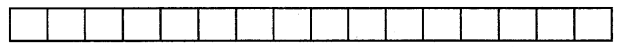Question 31.
Write the syntax for continue statement.
The syntax for continue statement is
—————
—————
continue;
—————Question 32.
What is an array?
Array is an ordered list of similar data elements.

Question 33.
What is C function?
C function is a set of instructions to carry out a particular task. (sqrt(), sin(), pow()).

Question 34.
What is the use of a function conio.h?
“conio.h” is a C header file used mostly by MS-DOS compilers to provide console input/ output.

### 2nd PUC Electronics C Programming Two Marks Questions and Answers

Question 1.
Write the commonly used directive.
# include<stdio.h> and #define pi 3.1415.

Question 2.
What does #include directive specify?
#include directive specifies an external file containing functions or macro definitions which can be included as a part of a program.
#include<stdio.h> includes standard function to perform input and output operations.Question 3.
Briefly explain #define directive.
#define directive is a compiler directive and not a statement. If this statement is included in the -program at the beginning, then the pre-processor replaces every occurrence of the identifier in the source code by the constant/string. For example if the statement is #define rate 20 then in the program whenever variable rate is considered then it is always assigned with constant 20.

Question 4.
Briefly explain #include directive.
#include directive specifies an external file containing functions or macro definitions which can be included as a part of a program.
#include<stdio.h> includes standard function to perform input and output operations.

Question 5.
Write the steps involved in executing a C program.
Steps involved in executing a C program are

• creating a program
• compiling the program
• linking the program with functions needed from C library and finally
• executing the program.

Question 6.
What are the different types of errors a programmer do?
Generally, programmers do three types of errors namely syntax error, logical error and run time error.

Question 7.
Briefly explain syntax error.
Syntax error is the result of violating the grammar of C programming language. When these errors occur, computer display an error message specifying the line number where the error has occurred. In the program segment
int a,b,c
a=20, b=c; computer will indicate semicolon is missing.

Question 8.
Briefly explain logical error.
As the name implies Logical errors are related to the logic of the program execution. Logical errors do not show compiler generated error messages rather they cause wrong results. These errors are primarily due to poor understanding the problem, incorrect translation of the algorithm into program and lack of clarity of hierarchy of operators. In the statement if(x= =y) printf(“They are equal\n”); when x and y are float type, they rarely become equal due to truncation errors. The printf may not be executed at all. Similarly test condition while(x!=y) might create an infinite loop.

Question 9.
Briefly explain run time error.
When the user tries to run ambiguous instructions run time error occurs. Errors of mismatching the data types, referencing out of range array element cannot be detected by the compiler. Divide by zero, data overflow are some examples.Question 10.
Mention the steps involved in running the program.
The steps to run the program are

• understand the instructions
• store data and instructions and
• perform computations.

Question 11.
For what purpose character sets are used?
Character sets are used to form words, numbers and expressions.

Question 12.
Write any four character sets of C.
The character sets of C are letters, digits, special characters and white spaces.

Question 13.
Mention the different C tokens.
Keywords, identifiers, constants, strings, operators and special symbols are the different C tokens.

Question 14.
What is a floating constant? Give an example.
Floating constant is a number with a decimal point. It is a sequence of digits preceded and followed by a decimal point. General form of floating constant is sign integer part decimal point fractional part. Example is 28.75, -0.00002345, 0.123e5.

Question 15.
What is a character constant? Give an example.
Character constant is a single character enclosed within a pair of apostrophes, ‘x’,’?’, ‘D’ etc.

Question 16.
What is a string constant? Give an example.
String constant is a sequence of characters enclosed within a pair of double quotes. “Hello”, “Pre-University”, “IIPUC ELECTRONICS”, “2014”, “ASCII”.Question 17.
What are variables? Give an example.
The quantity that changes during the execution of a program is a variable. Variables are the names given to identify the specific program elements. Variables are also called identifiers. Sum, height, mass, total, time etc.

Question 18.
Give an example each for valid variables and invalid variables.
Valid variables: total, area_triangle, net_salary etc. For invalid variables : 2total, area-triangle, net salary etc.

Question 19.
What are backslash costants?
Backslash constants are combination of two characters in which first character is always a back slash (\) and the second can be any one of the characters a,b,f,n,r,t,v, “, \, 0. These are also called escape sequences.

Question 20.
Write the C equivalent expression for (2x+1)(3y+2z)
(2*x+1)*(3*y+2*z)

Question 21.
Write any four arithmetic operators and their meaning.

• + addition or unary plus,
• – subtraction or unary minus,
• * multiplication,
• / division,
• % modulo division.

Question 22.
What is the meaning of a%b? Find the value of 21%4.
a%b always results in the remainder of division a/b. The value of 21%4 is 1.

Question 23.
What is the use of logical operator?
Logical operators are used to make decisions The results of these operations are either true or false.

Question 24.
If a=3, b=5 and c=6, find a && b || c || (!b) && (!c).
a=3, b=5 and c=6, a= 011, b=101 , c=110. a && b || c ||(!b) && (!c): (011&&101)||(110)||(010)& &(001) = 0011| 110||000 = 111= 7.Question 25.
What is data type conversion? Explain.
Changing the data type of the variables is called data type conversion or type casting.

Question 26.
Write the general format of ‘scanf( )’ formatted input.
General format of scanf( ) formatted input is scanf(“control string”, address list);

Question 27.
What should be the statement for an output displayed as 5.8628e-03? Write its value.
For displaying the output 5.8628e-03 the statement is x=0.0058628 printf(“%e”,x);

Question 28.
Mention different conditional control statements.
Different conditional statements are if statement, if-else, nested if-else and switch statement.

Question 29.
For what purpose if- statement and if-else statements are used?
If statement is used to execute a statement or a set of statements conditionally. It is also called one-way branching. If-else is used when there are two statements which are to be executed – alternatively. It is a two way branching.

Question 31.
Write the syntax of if statement.
The syntax of if statement is
if(condition)
{
Statement;
}Question 32.
Write the syntax of if-else statement.
The syntax of if-else statement is
if(condition)
{
Statementl;
}
else
{
Statement2;
}

Question 33.
Write the syntax of do while statement.
The syntax of do while statement is
do
{
Statement;
}
while(logexp);

Question 34.
Write the syntax of for- statement.
The syntax of for statement is
for (expression 1 ;expression2;expression3)
{
Statement;
}

Question 35.
What is use of break statement? Write its syntax.
Break statement is used to terminate the loop and exit from a particular switch case label. Syntax for break is

———————
———————
break;
———————-Question 36.
What are the rules used while writing subscripts?
Rules for subscripts are

• each subscript must be an unsigned positive integer constant or an expression
• subscript of a subscript is not permitted
• maximum subscript appearing in a program for a subscripted variable should not exceed the declared one
• subscript value ranges from 0 to one less than the maximum size. If an array has three elements then subscript can be 0,1,2.

Question 37.
Write a C program to find the product of two matrices and print the product matrix.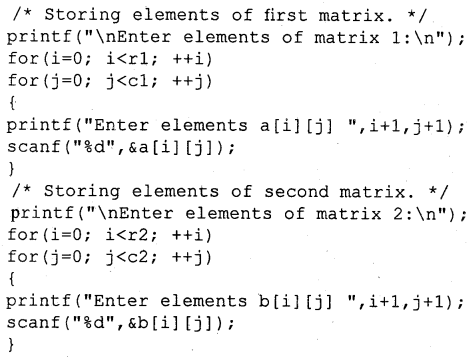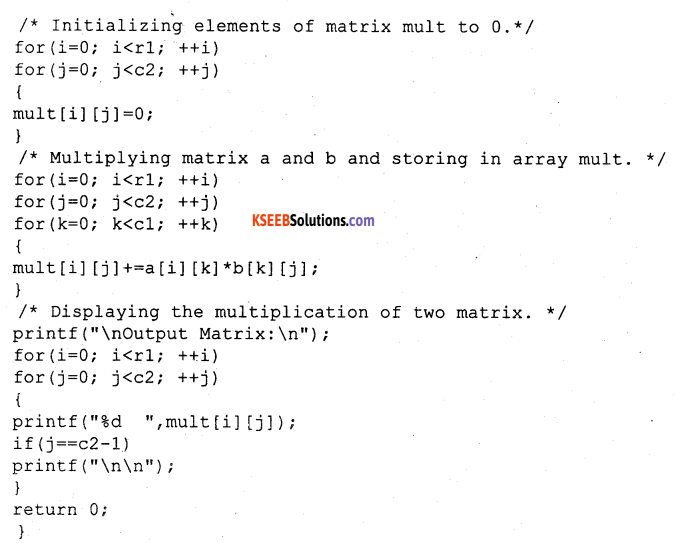Output
Enter rows and column for first matrix; 2
3
Enter rows and column for second matrix; 2
3
Error? column of first matrix not equal to equal to row of second. Enter rows and column for first matrix; 2
2
Enter elements of matrix 1:
Enter elements all;3
Enter elements a12:-2
Enter elements a13 : 5
Enter elements a21 : 3
Enter elements a22 : o
Enter elements a23:4

Enter elements of matrix 2:
Enter elements bn:2
Enter elements bn2:3
Enter elements b2n:-9
Enter elements b22:o
Enter elements b31:o
Enter elements b32:4

Output matrix:
24 29
6 25

### 2nd PUC Electronics C Programming Three Marks Questions and Answers

Question 1.
Mention the different sections that appear in C programming.
Documentation section, link section, definition section, Global declaration section, Function section, subgroup Section are the different sections that appear in C programming.

Question 2.
Write the format of simple C program.
Format of a simple C program is
main ( )
{
—————-
Statement;
—————-
}Question 3.
Briefly explain how to write a C program.
In writing a C program one style should be used and there should be consistency. Lower case letters are used to write a program. Group program statements are written together. Braces used to mark the beginning and end of the function. C is free from language, one can group statements together on same line. However, this make the program more difficult to understand.

Question 4.
Mention the different types of run time errors.
The run time errors are infinite loop in a program, divide by zero, null pointer assignment, data overflow.

Question 5.
Write the different constants used in a C program.
The constants used in C are broadly divided as numeric and non numeric constants. Numeric is further divided as integer and float point constant, while non numeric is divided as character and string constants.

Question 6.
Name any six operations.
Arithmetic, relational, logical, assignment, increment/decrement, conditional, bitwise, special operators.

Question 7.
Mention the different relational operators and their meaning.

 Operator Meaning < Lesser than <= Less than or equal to > Greater than >= Greater than or equal to = = Equal to != Not equal to

Question 8.
Write a C program to check whether the given two numbers are equal.Question 9.
Write a C program to compare two values.Question 10.
How do you represent logical AND, OR and NOT operators?
Logical AND is represented as &&, Logical OR as || and NOT as!

Question 11.
Briefly explain bitwise operators.
Bitwise operator works on bits and perform bit by bit operation. Consider a=5 and b=7 bitwise OR is 01011|0111=0111. For 1010 its l’s complement is 0101.

Question 12.
For an 8-bit computer A=6, B=7, find bitwise ANDing of A and B and bitwise ORing of A and B.
In an 8- bit computer, A=6, B=7. Bitwise ANDing of 00000110 &000GQ111 is 00000111. Bitwise ORing of 00000110 | 00000111 is 00000111.Question 13.
Find the bitwise complement of A=00001001, exclusive ORing of A=00101010 and B=10101100.
Bitwise complement of A=00001001 is 11110110 and exclusive OR of 00101010 and 10101100 is 10000110.

Question 14.
Write a C program to illustrate the compact representation of arithmetic operations.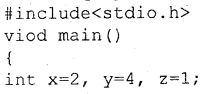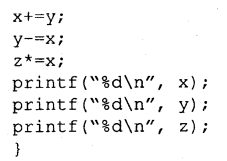Question 15.
Write a C program to illustrate the use of increment operator.Question 16.
Briefly explain operator precedence in C.
Operators precedence determines the grouping of terms in an expression. This affects how an expression is evaluated. Certain operators have higher precedence than the others. For example, x= 6+2*4; in this expression x is assigned 14 but not 32, this is because * has higher precedence compared to +.

Question 17.
Which are the input and output functions used in C?
To perform input-output operations C provides a library functions. This is called standard input-output library. It is denoted as stdio. Header file containing such library functions is stdio.h. scanf( ), printf( ), getchar( ), putchar( ), gets( ), puts( ), getch( ),
getche ( ) are the standard functions in C performing i/o operations.

Question 18.
Write the formatted and unformatted i/o functions in C.
Function scanf( ) is used to read the values for the variables in C from the keyboard. The scanf( ) is used to enter the numeric, character and string type data. It is included in the header file <stdio.h>. C programming provides printf( ) function to display the data on the output device(monitor). Syntax for scanf( ) and printf( ) is printf(“control string”, variable); scanf(“control string”, address_list);

Question 19.
Mention the different character groups and their meaning.

Question 20.
How does the monitor display 321 for statement
i) printf(“%d”,x);
ii) printf(“%d”,x);
i) 321
ii) 321

Question 21.
How does the monitor display 28.689 for statement
i) printf (“%5.3f”,p);
ii) printf(“%e”,y);
i) 28.689
ii) 2.8689e+01Question 22.
Write the display format for the statements
i) printf (“%19.10s”book); Pre-University
ii) printf(“% -21.10s”,book); s-Pre-University
i) In this print statement 10 characters are right justified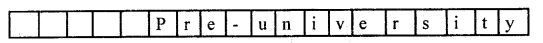ii) In this print statement 10 characters are left justifiedQuestion 23.
Draw a flow chart for if-else statementQuestion 24.
Mention the different control statements.
There are two control statements namely conditional control and goto statement respectively. Conditional control further consists of if statement, if-else statement, nested-if statement and switch statement.

Question 25.
Draw a flow chart for if-statement.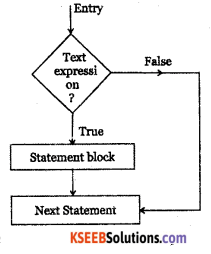Question 26.
Write the syntax for nested- if statement.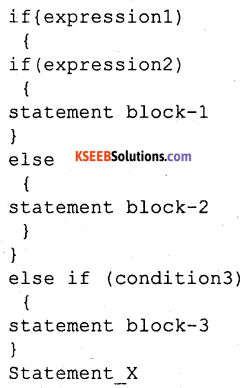Question 27.
Briefly explain loop control statement.
Program loop consists of two segments

• Body of the loop and
• Control statement.

The test condition should be carefully stated in order to perform the desired number of loop execution. Test condition should transfer the control out of the loop as the condition false other wise control sets up an infinite loop. A looping process would include Setting & initialization of a counter, Execution of the statement in the loop, Test for the specified condition for execution of the loop and Updating the counter.

The C language provides three loop constructs for performing loop operation The While statement, The do-while statement and The for statement.Question 28.
Draw a flow chart for while control statement.Question 29.
Write the syntax for multi dimentional arrays.
Type array name [sizel] [size] [size3]…………. [size n];

### 2nd PUC Electronics C Programming Four Marks Questions and Answers

Question 1.
Write the features of C.
C is a powerful, flexible language that has gained worldwide acceptance in recent years. C is concise, yet powerful in its scope. It is a high-level language that also incorporates features of low-level languages. It is becoming the standard for program development on small machines and microcomputers.

It is possible to apply C to nearly all application areas. Modularity {process of dividing problems into sub-problems) makes C ideal for projects involving several programmers. C permits nearly every programming technique. C is widely available for most brands and type of computers. It is portable. With all the features C is not a difficult language, it is one of the easiest languages to learn and implement.

C programming can be used to write any complex program because of its rich set of built-in functions and operations. C compiler combines the capabilities of an assembly language with the feature of high-level language and therefore it is very much suited for uniting both system software and business packages. Because of its variety of data types, powerful operator programs in C are efficient and fast. C has the ability to extend, it means user-defined functions can also be added to C library.

Question 2.
Write ten basic structure of C programming (overview).
C program may contain one or more sections as shown in fig. 1.
Overview of a C program

 Documentation Section Link Section Definition Section Global declaration Section main( ) Function Section { Declaration part Executable part } Subprogram Section Function 1 Function 2 Function 3 Function 4 ………………… (User-defined functions) Function n

Question 3.
Briefly explain the format of a simple C program.
Every C program you create includes the following components
Pre-processor directives
main( )
A pair of flower brackets { }
Declarations and statements
User created sub-programs or functions
{
Declarations and statements
}
User created sub-programs or functions
The above-described components are organized as follows
Pre-processor directives
main( )
{
Declarations and statements
}
User created sub-programs or functions

Pre-processor Directives

A C program contains one or more functions or sub programs. Function is a series of instructions to the computer to perform a specific task. Many functions are already written and compiled in the library supplied. Instead of writing the individual instructions user can tell the compiler to use one of its standard functions. Identifying the class of functions to be used is done in the pre-processor directive.
#include <stdio.h>
#include <string.h>
are some examples of pre-processor directives.
main ( )
Every C program must begin with the function called main( ), which is the identification for the start of the main part of the program and it looks just like main( ).
The parentheses after main( ) are part of the function name and are necessary. They tell the compiler that function is referring to and not the word main. A pair of parentheses must follow every function.

A pair of flower brackets { }

Following main( ) are declarations and statements. An opening brace must appear immediately after the word main( ) and closing brace “}” must follow the last instruction. The flower brackets or braces thus indicate the body of the program, which includes instructions to perform the required task.

Declarations and Statements

The declarations represent that part of the program where all the variables, arrays, functions and so on are identified with their data types. Sometimes the various elements are initialized in this part. The statements can include instructions to perform certain operations such as input or output or assignment. Statements can also include C commands or the names of functions in the library.Question 4.
Draw the flowchart to show the process of compiling and running C program.
Flowchart is shown in fig. 1.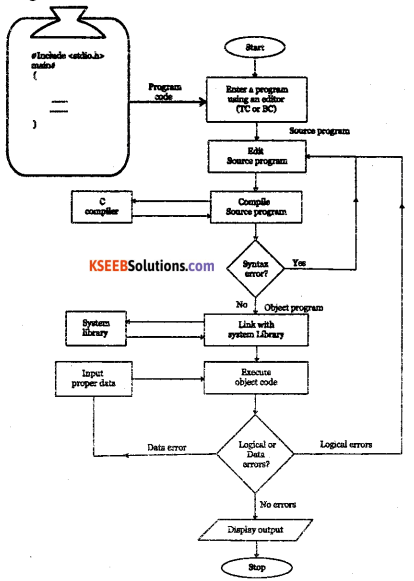Question 5.
Write the rules for forming the identifier name.
Rules to be followed while creating identifiers:

1. The first character of an identifier should always start with an alphabet or an underscore, which can be followed by either alphabets or digits or certain symbols.
2. An identifier may be of any length up to 32 characters. Some compilers limit the length to eight characters.
3. Identifiers can contain both uppercase and lowercase letters. C is case sensitive, that is, lowercase letters are not converted to uppercase letters when used in identifiers.
4. Special characters except underscore should not be used in identifiers. Successive underscores are also not allowed.
5. Identifiers should be single words i.e., blank spaces cannot be included in identifiers.
6. Reserved words should not be used as identifiers.
 Legal identifiers Illegal identifier Legal but poor Sum Emp_name Student_address Number age Varun’s_addr while @total sum do-it counter 3by2 _help sumo_1

Question 6.
Write the rules for forming a variable name.

• Variable name consists of Setters, digits & underscore (_)
• Must begin with a letter. Some systems permit an underscore as the first character.
• ANSI standard recognizes a length of 31 characters. However, the length should not be normally more than 8 characters, since only the first eight characters are treated significant by the compiler.
• Uppercase and lowercase are significant, ie TOTAL is not same as total or
• Variable name should not be a keyword.
• White space is not allowed.

Example: Valid: temp, avg, suml ,total, ph value, T_raise, mark, i, j Invalid: 123,25th 5times, (area) abc\$ char, int, group one abc-5Question 7.
If a=25 and b=6, find
(i) a+b
(ii) a – b
(iii) a * b
(iv) a/b
(v) a%b
(vi) -a%b
(vii) a%-b
a=25 and b = 6.
(i) a+b = 25+6 = 31
(ii) a-b = 25-6 = 19
(iii) a*b= 25*6 =150
(iv) a/b=25/6 = 4
(v) a%b=25%6 = 1
(vi) -a%b= -25%6 = -1
(vii) a% -b= 25% -6 = 1

Question 8.
Write the C program to convert the given number of days into months and days. Enter days as 196 days.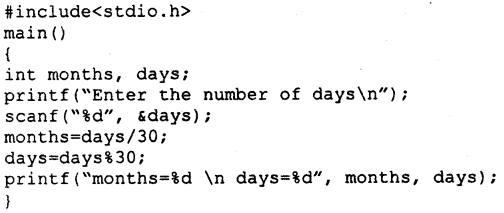Output:
Enter the number of days 196
months = 6
days= 16

Question 9.
Write the C program to accept three numbers and compute their sum and average. Find the sum and average of 3,7 and 8.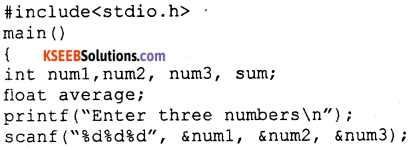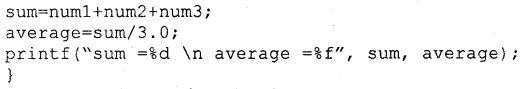Output:
Enter three numbers 3 7 8
sum -18
average = 6.000000

Question 10.
Write the C program to accept the radius of a circle and compute its area and perimeter. Radius, r = 7m.Output:
Area of the circle = 153.957993m
Perimeter = 43.987999m

Question 11.
Write the C program to accept two floating point numbers and compute their ratio. If the ratio is greater than zero then it should exchange the contents of the numbers.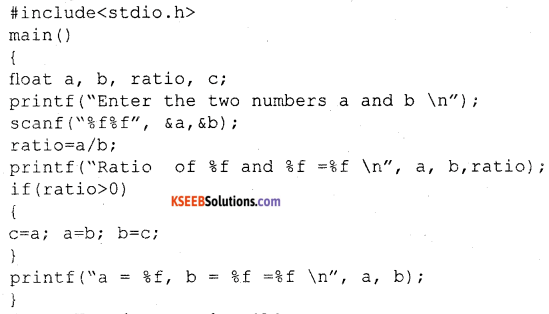Output:
Enter the two numbers 18.0
24.0
Ratio = 0.750000
a = 24.000000,
b=18.000000

Question 12.
Write the C program to accept two numbers and check whether they are equal or unequal.Output:
Enter the two numbers
15
24
15 and 24 are NOT equal
Enter the two numbers
25
25
25 and 25 are equal

Question 13.
Write the C program to accept three numbers and print the largest amongst them.Output:
Enter the three numbers 15
24
48
48 is largest number among 15,24 and 48Question 14.
Write the C program to find the roots of a quadratic equation ax2 + bx + c = 0 using switch statement.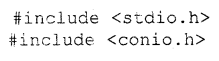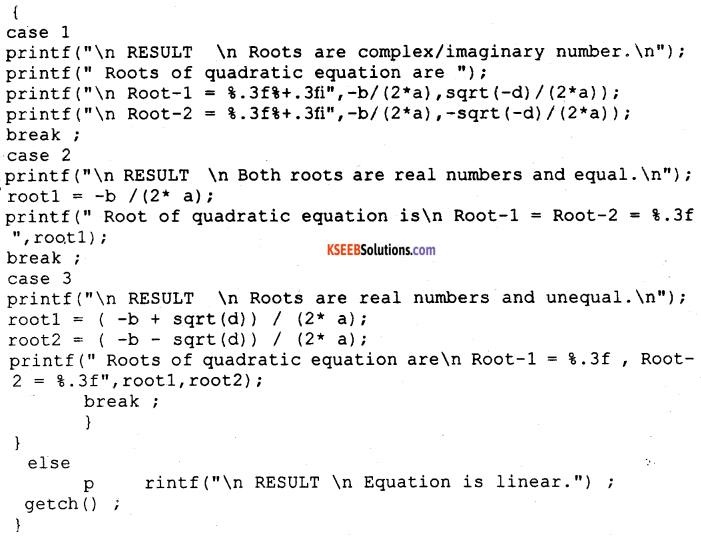Output:
Enter the values of A; 1 Enter the values of B : 3 Enter the values of C: 8 RESULT:
Roots are complex/imaginaiy number. Roots of quadratic equation are:
Root-1 -1.500*2.398!
Root-2 -1.500-2.398i
Enter the values of A: 2 Enter the values of B : 4 Enter the values of C: 2 RESULT:
Both roots are real numbers and equal. Root of quadratic equation is:
Root-1 = Root-2 = -1.000
Enter the values of A: o Enter the values of B : 5 Eater the values of C : 8 RESULT:
Equation is linear.
Eater the values of A: 3 Enter the values of B : 8 Enter the values of C : 2 RESULT:
Roots are real numbers and unequal. Roots of quadratic equation are: Root-i = -0.279 , Root-2 = -2.387

Question 15.
Write the C program to print the sum of all even integers between 1 and 55.Output:
sum of all even numbers between 1 and 55 is 756

Question 16.
Write the C program to print the sum of the first 89 natural numbers.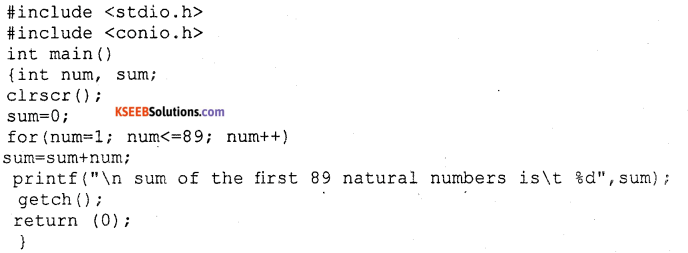Output:
sum of the first 89 natural numbers is 4005Question 17.
Write the C program to accept n integers and store them in an array called num and also print them.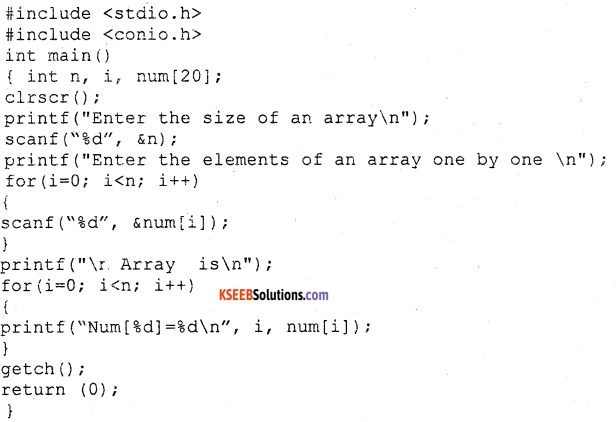Output:
Enter the size of an array
3
Enter the elements of an array one by one
10
22
13
Array is
Num = 10
Num =22
Num  = 13

Question 18.
Write the C program to accept the integer arrays and find the sum of the corresponding elements of the two arrays.Output:
Enter the size of the arrays A and B 3
Enter the elements of the array A one by one
10
22
13
Enter the elements of the array B one by one
10
22
13
Sum of elements of A and B 20 44 26Question 19.
Write the C program to accept two matrices of the same order and find the sum of the corresponding elements of the matrices and print the sum matrix.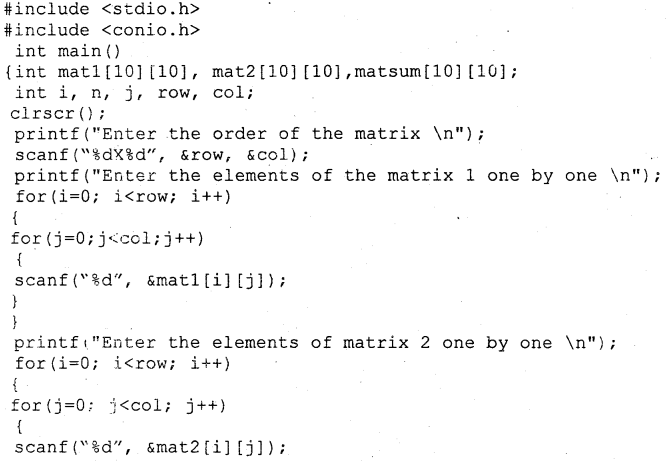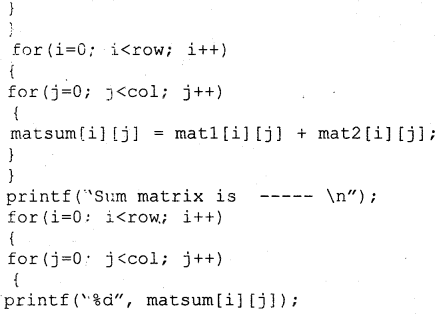Output:
Enter the order of the matrix .2 X 2
Enter the elements of the matrix 1 one by one 10
20
30
40
Enter the elements of the matrix2 one by one 10
20
30
40
Sum matrix is——
20
40
60
80

error: Content is protected !!